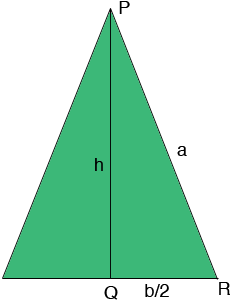SEARCH HOMEMath Central Quandaries & QueriesQuestion from Brian: how can I find the base of an isosceles triangle from the height and the perimeter?Hi Brian,

Suppose the base has length b, each of the other two sides has length a, the perimeter is p and the height is h. Let Q be the midpoint of the base and draw a line from Q to the apex of the triangle P.By the symmetry angle PQR is a right angle and thus the Theorem of Pythagoras applies so that

a2 = h2 + (b/2)2.

You also know that

2a + b = p.

Use these equations to find an expression for b in terms of p and h.

PennyMath Central is supported by the University of Regina and The Pacific Institute for the Mathematical Sciences.